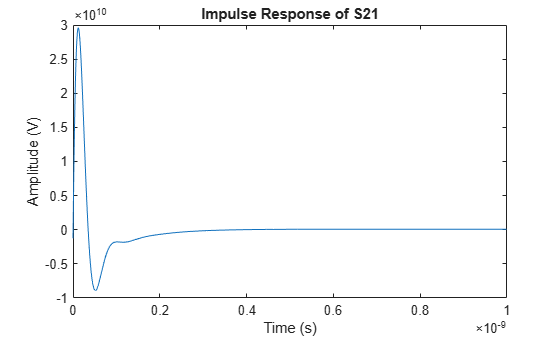impulse

Impulse response for rational function object

Description

example

[response,tout] = impulse(h,ts,n) computes the impulse response of a rational function object, h, over a time period specified by ts and the number of samples n.

Note

While you can compute the output response for a rational function object by computing the impulse response of the object and then convolving that response with the input signal, this approach is not recommended. Instead, you should use the timeresp method to perform this computation because it generally gives a more accurate output signal for a given input signal.

Examples

collapse all

Create a sparameters object from a file and use rfparam to extract the ${\mathit{S}}_{21}$parameters.

S = sparameters('passive.s2p');
S21 = rfparam(S,2,1);

Fit a rational function object to the ${\mathit{S}}_{21}$ data by using rationalfit.

freq = S.Frequencies;
fit_data = rationalfit(freq,S21)
fit_data =
rfmodel.rational with properties:

A: [6x1 double]
C: [6x1 double]
D: 0
Delay: 0
Name: 'Rational Function'

Calculate the impulse response using the impulse method and plot the results.

[resp,t] = impulse(fit_data,1e-12,1e3);
plot(t,resp);Input Arguments

collapse all

Rational function object, specified as a rationalfit object handle.

Data Types: double
Complex Number Support: Yes

Sample time of the computed impulse response, specified as a positive scalar integer in seconds.

Data Types: double

Number of samples, specified as a positive scalar integer.

Data Types: double

Output Arguments

collapse all

Impulse response, returned as an n element vector of impulse response values.

Sample time of the output signal, returned as a positive scalar integer in seconds.

Data Types: double

collapse all

Impulse Response And Time Samples Equation

RF Toolbox™ uses the following equation to for the impulse response:

$resp=\sum _{k=1}^{M}{C}_{k}{e}^{{A}_{k}\left(t-Delay\right)}u\left(t-Delay\right)+D\delta \left(t-Delay\right)$

where

• A, C, D, and Delay are properties of the rational function object, h.

• M is the number of poles in the rational function object.

The vector of time samples of the impulse response, t, is computed from the inputs as t = [0,ts,2*ts,...,(n-1)*ts]